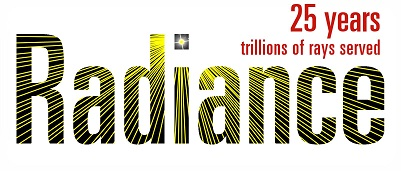# Modeling Photochromic glass using Radiance

Hello everyone,

We want to simulate a red photochromic glass using Radiance. The UV-VIS spectroscopy analysis was conducted and optical characteristics of the red glass including transmittance and absorbance were recognized. Then, the transmissivity of the glass sample was calculated based on the following formula.

Transmissivity = (sqrt (0.8402528435 + 0.0072522239 * Transmittance ^ 2) - 0.9166530661) / 0.0036261119 / Transmittance)

For example, the Transmittance of red glass is 48 % and the transmissivity is 0.5232.

We can model the red photochromic glass in three different ways in Radiance. I appreciate if you clarify which one is correct.

1-First method

Void glass Red_Transmittance_48

0

0

3 0.5232 0.5232 0.5232

2-Second method

Void glass Red_Transmittance_48

0

0

3 0.5232 0 0

Note: In the second way, we remove the parameters of gtn and btn because of red color of the sample.

3- Third method

Void glass Red_Transmittance_48
0
0
3 0.211 0.086 0.196

Note: Some scholars suggested to add the wavelengths of lights (red, green, blue) in the definition of glass material in Radiance. Because of the color of glass, we consider the wavelengths of lights (red, green, blue) in transmissivity calculations and then in the definition of glass material in Radiance.

Among these three ways, which one is the correct way of modeling photochoromic glass in Radiance?